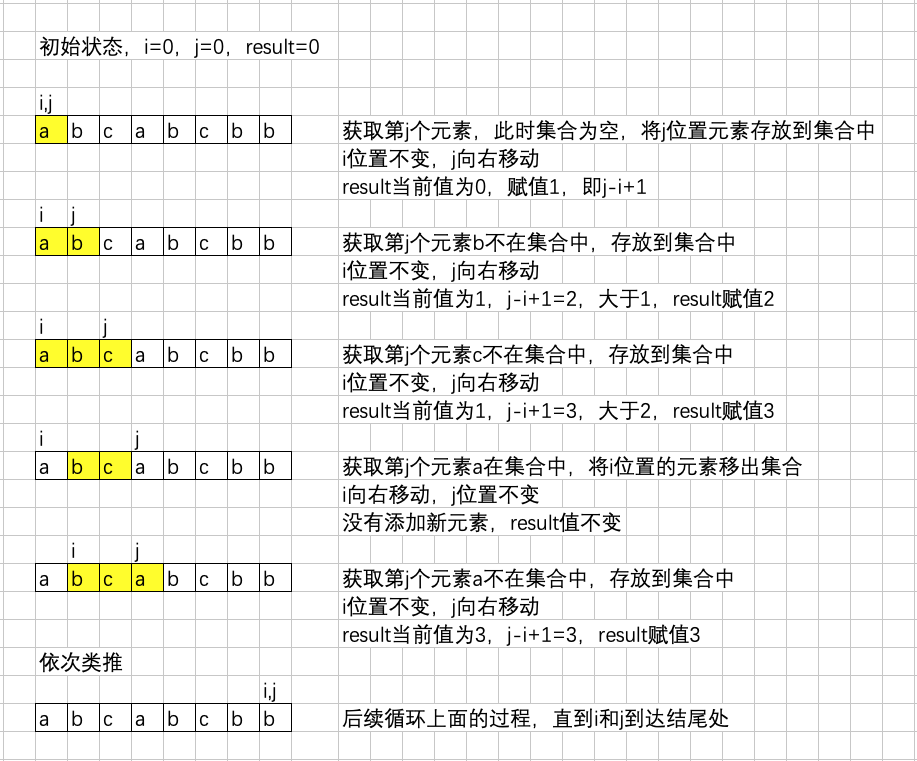``````import java.util.HashSet;
import java.util.Set;

class Solution {
public static int lengthOfLongestSubstring(String s) {
if (s == null || s.isEmpty()) {
return 0;
}
final int len = s.length();
final Set<Character> sets = new HashSet<>();
int i = 0, j = 0, result = 0;
Character tmp ;
while (i < len && j < len) {
tmp = s.charAt(j);
if (sets.contains(tmp)) {
sets.remove(s.charAt(i++));
} else {
result = Math.max(result, j++ - i + 1);
}
}
return result;
}
}``````

• 对于数组，指两个变量在数组上相向移动解决的问题；
• 对于链表，指两个变量在链表上同向移动解决的问题，也称为「快慢指针」问题。

CSDN 主页：http://blog.csdn.net/liuxinghao
CSDN 博文：面试真题：无重复字符的最长子串